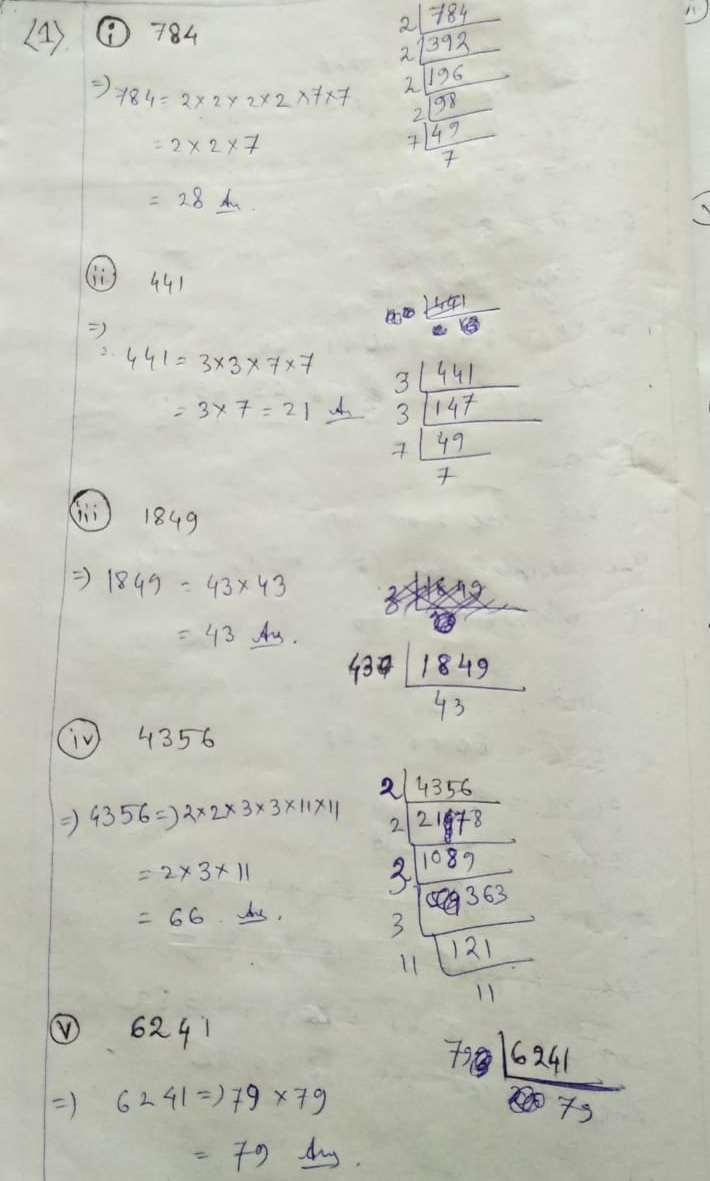# ML Aggarwal CBSE Solutions Class 8 Math Third Chapter Squares and Square Roots Exercise 3.3

### ML Aggarwal CBSE Solutions Class 8 Math 3rd Chapter Squares and Square Roots Exercise 3.3∴ 6 should be multiplied to get a perfect square

∴ 294 x 6 = 1764 = 6 x 6 x 7 x 7

∴ 1764 is perfect square root.

√1764 = √6x6x7x7 = 6 x 7

= 42

∴ square root is 42∵ 3 is unpaired

∴ 3 should be divided

∴ The perfect sq root = 613747/3

= √20449 = 143(7) If 64 student does not accommodated

Then, the num of row = the num of column

No of students arrange = 2000

∴ If 64 student could not accommodated,

then no of students = 2000 – 64

= 1936

∴ No of row = √1936 = No of columns

= 2 x 2 x 2 x 11 x 11

= 2 x 2 x 11

= 44

(8) According to question

No. of students = No of contributed rupee

Total rupees Rs. 2304

∴ No of students = √2304

= 2 x 2 x 2 x 2 x 12 x 12

= 2 x 2 x 12

= 48

(9) One number = x

another number = 15x

According to the question

15x X x = 7260

Or, 15x2 = 7260

Or, x2 = 7260/15

Or, x = √484

= √2x2x11x11

= 2 x 11

= 22

One number = 22

Another number = 22 x 15 = 330

(10) Common factor = (2+3+5) 10

1st positive number = 2/10 x 950

190

2nd positive number = 3/10 x 950

285

3rd positive number = 5/10 x 950

= 475

(11) 1st sq’s perimeter = 60m = 4L

∴ 1st sq’s one side = L1 = 60/4 = 15 m

∴ 1st sq’s area = 15 x 15 = 225 m2

2nd sq’s perimeter = 144 m = 4 L

∴ 2nd sq’s one side = L2 = 144/4 = 36

∴ 2nd sq’s area = 36 x 26 = 1296 m2

Sum of area of 1st & 2nd sq = (225 + 1296) m2

= 1521 m2

∴ According to question –

3rd sq’s area = 1521 m2

∴ Let, 3rd sq’s area = L32

∴ L32 = 1521

Or, L3 = √1521 = 39

∴ 3rd sq’s perimeter = 4L = 39 x 4

156 mUpdated: February 15, 2021 — 10:46 pm